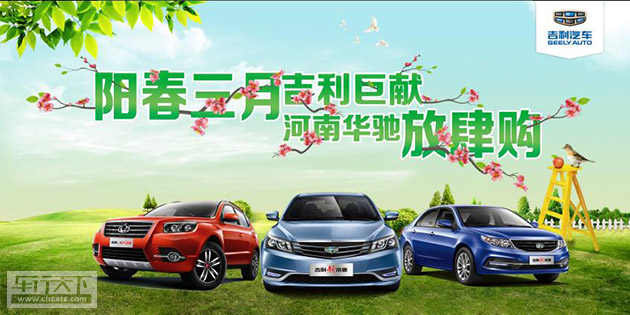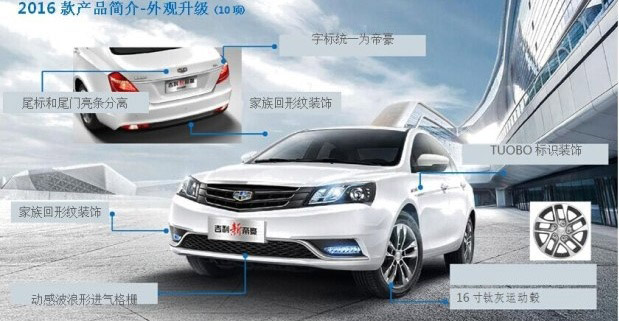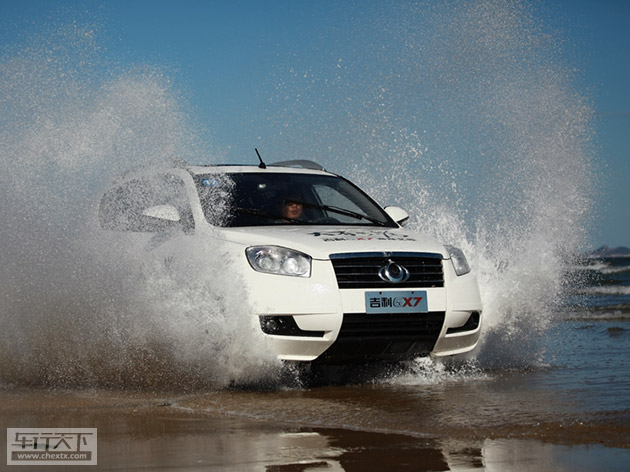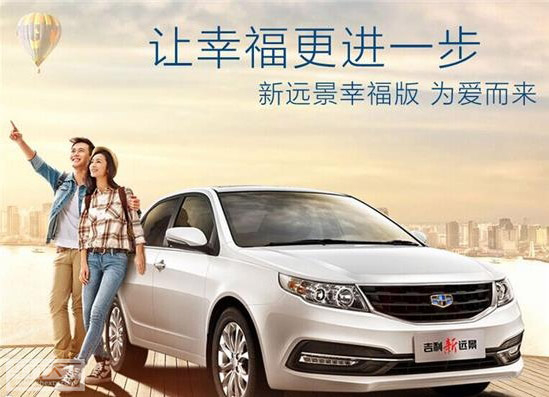## 阳春三月吉利钜献 河南华驰放肆购

2016年03月17日 16:05 来源：www.chextx.com 超过：次关注

什么时候买车最合适，当然是3月买车嘛！
话说：三月不买车，四月徒伤悲！

3月不是一个普通的月份，它代表着整个季度！3月买车便宜！

3月天气好，适合看车，更适合买车！物流方面也不会受到影响，大部分车型都会有现车。3月买车有现车！
3月买车，4月磨合，5月上高速全家出远门旅行！爽歪歪！

吉利三月巨献  河南华驰三月狂欢放肆购，给您送爱车，没错！您没看错！就是给您送爱车！！！河南华驰与厂家一起放肆卖车，货源充足，现场挑车试驾！
吉利新帝豪百万销量一路向上T动力现金钜惠10000元，远景十年庆，幸福百万家，工厂感恩综合钜惠6000元，吉利GX7直降万元购置税全减，1元就包牌，绝对的物超所值，就等您来，爱车送给您！活动时间：3月20日（本周日）

活动地点：中州大道河南华驰店（河南华驰新郑直营店）
活动内容：

1、吉利新帝豪  百万销量  一路向上   T动力现金钜惠10000元

2、远景十年庆、幸福百万家、工厂感恩综合钜惠6000元
3、吉利GX7直降万元购置税全减，1元就包牌，河南华驰GX7全面清库，最高现金直降15000元

机会仅此一次 、机不可失，失不再来！ 机会留给有实际行动的人！！！

试驾礼：当天试驾任意车型均可领取神秘礼品一份；

购车礼：到店可抽取购车红包，最高千元，可当天购车抵用；
金融礼：低首付，低利息，吉利金融更实惠，再送行车记录仪；
友情礼：带朋友来店提车，成功赠送常规保养1次；
换新礼：任意车型置换吉利汽车均可享受1000-5000元不等置换补贴；
惊喜礼：活动当天订购任意车型均可参与砸金蛋，蛋蛋有礼，喜上加喜！

没错！购任意车型再送888元的行车记录仪

3月20日，河南华驰吉利汽车4S店中州大道店不堵车的旗舰店与您不见不散！！2016款新帝豪性价比更高配置更丰富 帝豪RS性价比更高配置更丰富并富有运动性，郑州河南华驰独家授权！购吉利GX7直降万元购置税全减，1元就包牌，现有一批库存车出厂价处理，绝对低价，就等你来！吉利新远景为爱而来让幸福更近一步

省事：一站式搞定您的选车、购车、保险、分期、上牌，提供专业的顾问式购车服务。

省时：紧邻中州大道交通便利不堵车；合格证出证快上牌不担忧；足不出户即可选购你的爱车！
省心：价格一步到位！一降到底！
购车贷款更加“方便、快捷”！
“省心、放心”的一站式保险管家服务
“专业、可靠”的维修保养服务QQ：1826041575  625252307

#### 相关文章

﻿
• 快速找车
• 选择品牌
• 选择品牌
• A  奥迪
• A  阿斯顿·马丁
• A  阿尔法·罗密欧
• B  宝沃
• B  布加迪
• B  巴博斯
• B  保时捷
• B  宾利
• B  奔驰
• B  宝马
• B  本田
• B  别克
• B  标致
• B  比亚迪
• B  宝骏
• B  北汽制造
• B  北汽新能源
• B  北汽幻速
• B  北汽威旺
• B  北京汽车
• B  奔腾
• B  北汽绅宝
• B  北汽昌河
• C  长安欧尚
• C  长安
• C  长安凯程
• C  长城
• D  大众
• D  道奇
• D  DS
• D  东南
• D  东风风神
• D  东风风行
• D  东风小康
• D  东风风度
• D  东风
• F  福特
• F  丰田
• F  菲亚特
• F  法拉利
• F  福田
• F  福迪
• F  福汽启腾
• G  观致
• G  广汽传祺
• G  广汽吉奥
• G  GMC
• H  红旗
• H  汉腾汽车
• H  哈弗
• H  哈飞
• H  海格
• H  海马
• H  华颂
• H  黄海
• H  华泰
• H  恒天
• J  捷途
• J  几何汽车
• J  捷达
• J  吉利汽车
• J  捷豹
• J  Jeep
• J  江淮
• J  江铃
• J  金杯
• J  九龙
• J  金旅
• K  凯翼
• K  凯迪拉克
• K  克莱斯勒
• K  科尼塞克
• K  卡威
• K  开瑞
• L  路虎
• L  林肯
• L  劳斯莱斯
• L  兰博基尼
• L  雷克萨斯
• L  铃木
• L  领克
• L  雷诺
• L  理念
• L  力帆
• L  莲花汽车
• L  猎豹
• L  路特斯
• L  陆风
• M  马自达
• M  MG
• M  MINI
• M  玛莎拉蒂
• M  摩根
• M  迈凯轮
• N  纳智捷
• O  欧拉
• O  欧宝
• O  讴歌
• O  欧朗
• Q  奇瑞
• Q  起亚
• Q  启辰
• R  日产
• R  荣威
• R  瑞麒
• S  SERES赛力斯
• S  三菱
• S  斯威汽车
• S  萨博
• S  smart
• S  斯柯达
• S  斯巴鲁
• S  思铭
• S  双龙
• S  上汽大通
• S  双环
• T  特斯拉
• T  腾势
• W  蔚来
• W  沃尔沃
• W  WEY
• W  五菱汽车
• W  五十铃
• W  威兹曼
• W  威麟
• X  现代
• X  雪佛兰
• X  星途
• X  雪铁龙
• X  小鹏汽车
• X  西雅特
• Y  一汽
• Y  英菲尼迪
• Y  英致
• Y  依维柯
• Y  野马汽车
• Y  永源
• Z  众泰
• Z  中华
• Z  中兴
• Z  知豆
• 选择车系
• 选择车系
• 车型对比
• 选择品牌
• 选择品牌
• A  奥迪
• A  阿斯顿·马丁
• A  阿尔法·罗密欧
• B  宝沃
• B  布加迪
• B  巴博斯
• B  保时捷
• B  宾利
• B  奔驰
• B  宝马
• B  本田
• B  别克
• B  标致
• B  比亚迪
• B  宝骏
• B  北汽制造
• B  北汽新能源
• B  北汽幻速
• B  北汽威旺
• B  北京汽车
• B  奔腾
• B  北汽绅宝
• B  北汽昌河
• C  长安欧尚
• C  长安
• C  长安凯程
• C  长城
• D  大众
• D  道奇
• D  DS
• D  东南
• D  东风风神
• D  东风风行
• D  东风小康
• D  东风风度
• D  东风
• F  福特
• F  丰田
• F  菲亚特
• F  法拉利
• F  福田
• F  福迪
• F  福汽启腾
• G  观致
• G  广汽传祺
• G  广汽吉奥
• G  GMC
• H  红旗
• H  汉腾汽车
• H  哈弗
• H  哈飞
• H  海格
• H  海马
• H  华颂
• H  黄海
• H  华泰
• H  恒天
• J  捷途
• J  几何汽车
• J  捷达
• J  吉利汽车
• J  捷豹
• J  Jeep
• J  江淮
• J  江铃
• J  金杯
• J  九龙
• J  金旅
• K  凯翼
• K  凯迪拉克
• K  克莱斯勒
• K  科尼塞克
• K  卡威
• K  开瑞
• L  路虎
• L  林肯
• L  劳斯莱斯
• L  兰博基尼
• L  雷克萨斯
• L  铃木
• L  领克
• L  雷诺
• L  理念
• L  力帆
• L  莲花汽车
• L  猎豹
• L  路特斯
• L  陆风
• M  马自达
• M  MG
• M  MINI
• M  玛莎拉蒂
• M  摩根
• M  迈凯轮
• N  纳智捷
• O  欧拉
• O  欧宝
• O  讴歌
• O  欧朗
• Q  奇瑞
• Q  起亚
• Q  启辰
• R  日产
• R  荣威
• R  瑞麒
• S  SERES赛力斯
• S  三菱
• S  斯威汽车
• S  萨博
• S  smart
• S  斯柯达
• S  斯巴鲁
• S  思铭
• S  双龙
• S  上汽大通
• S  双环
• T  特斯拉
• T  腾势
• W  蔚来
• W  沃尔沃
• W  WEY
• W  五菱汽车
• W  五十铃
• W  威兹曼
• W  威麟
• X  现代
• X  雪佛兰
• X  星途
• X  雪铁龙
• X  小鹏汽车
• X  西雅特
• Y  一汽
• Y  英菲尼迪
• Y  英致
• Y  依维柯
• Y  野马汽车
• Y  永源
• Z  众泰
• Z  中华
• Z  中兴
• Z  知豆
• 选择车系
• 选择车系
• 选择车型
• 选择车型
• 意见反馈